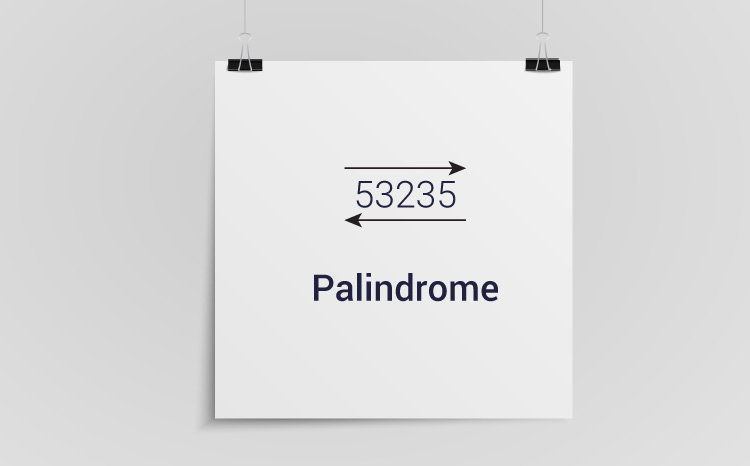# C Program to Check Whether a Number is Palindrome or Not

In this example, you will learn to check whether the number entered by the user is a palindrome or not.To understand this example, you should have the knowledge of the following C programming topics:

An integer is a palindrome if the reverse of that number is equal to the original number.

## Program to Check Palindrome

``````#include <stdio.h>
int main() {
int n, reversedN = 0, remainder, originalN;
printf("Enter an integer: ");
scanf("%d", &n);
originalN = n;

// reversed integer is stored in reversedN
while (n != 0) {
remainder = n % 10;
reversedN = reversedN * 10 + remainder;
n /= 10;
}

// palindrome if orignalN and reversedN are equal
if (originalN == reversedN)
printf("%d is a palindrome.", originalN);
else
printf("%d is not a palindrome.", originalN);

return 0;
}

``````

Output

```Enter an integer: 1001
1001 is a palindrome.
```

Here, the user is asked to enter an integer. The number is stored in variable n.

We then assigned this number to another variable orignalN. Then, the reverse of n is found and stored in reversedN.

If originalN is equal to reversedN, the number entered by the user is a palindrome,